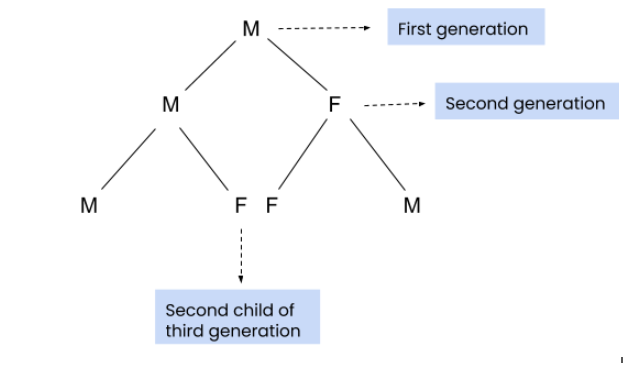# Family Structure

Posted: 16 Dec, 2020
Difficulty: Easy

## PROBLEM STATEMENT

#### Aakash is a member of Ninja club. He has a weird family structure. Every male member (M) gives birth to a male child first and then a female child, whereas every female (F) member gives birth to a female child first and then to a male child. Aakash analyses this pattern and wants to know what will be the Kth child in his Nth generation. Can you help him?

``````A sample generation tree is shown, where ‘M’ denotes the male member and ‘F’ denotes the female member.
``````##### Note
``````The generation tree starts with a male member i.e. Aakash.
Every member has exactly two children.
The given N and K will always be valid.
``````

#### Input Format:

``````The first line contains an integer 'T' which denotes the number of test cases. Then the test cases follow.
The first and the only line of each test case contains two space-separated integers ‘N’ and ‘K’ denoting the generation number and position of the child in Nth generation, respectively.
``````

#### Output Format

``````For each test case, print the gender of the Kth child in the Nth generation. If the gender is male, print “Male” else print “Female” (without quotes).
The output of each test case is printed in a separate line.
``````

#### Note

``````You don’t have to print anything, it has already been taken care of. Just implement the given function.
``````

#### Constraints :

``````1 <= T <= 5
1 <= N <= 50
1 <= K <= 2^(N - 1)
where ‘T’ is the number of test cases, ‘N’ is generation number and ‘K’ is the position of the child in the Nth generation.
``````Approach 1

The idea is to find the gender of the parent of the Kth child in Nth generation. Now, if the Kth child of the Nth generation is the first child of its parent, then it’s gender will be the same as the parent’s gender else it will be the opposite. This can be done recursively.

Algorithm:

• The idea is to use recursion.
• Base condition, If 'N' or 'K' is 1, return “Male”, as the first child of every generation is male.
• Find the parent of the Kth child of Nth generation. It will be floor((K + 1) / 2). Store it in a variable “par”.
• Note that the generation of the parent is “N - 1”. Thus, the parent will be the floor((K + 1) / 2)th child of (N - 1)th generation.
• Recursively find the gender of the parent and store it in a string "parGender". If the Kth child of Nth generation is the first child of its parent, i.e. if 'K' is equal to (2 * par - 1), return the gender of the parent ("genParent").
• Else, return the gender opposite of its parent’s gender. If its parent is “Male”, return “Female” else return “Male”.

For example: N = 3, K = 3

We need to find the gender of the 3rd child in the 3rd generation.

Now, par = (K + 1) / 2 = (3 + 1) / 2 and generation = N - 1 = 2.

Now, we have to find the parent of the second child of the second generation.

par = (2 + 1) / 2 = 1 and generation = 1.

As the generation is 1, simply return “Male”.

Now, we know the gender of the parent of the 2nd child of the 2nd generation. As the 2nd child of the 2nd generation is the second child of its parent, its gender will be Female(opposite of the parent).

Now, we know the gender of the parent of the 3rd child of the 3rd generation. As the 3rd child of the 3rd generation is the first child of its parent, its gender will be Female( same as the parent’s).

Thus, “Female” is the required answer.